# Electronics: Resistors in parallel

From relation for calculating the resistance of parallel combination of resistors:

$\frac{1}{R}=\frac{1}{{R}_{1}}+\frac{1}{{R}_{2}}$

Calculate the R, if R1 = 2Ω a R2 = 15Ω

R =  1.76 Ω

### Step-by-step explanation:

R = \dfrac{1}{ \dfrac{1}{ 2}+\dfrac{1}{ 15}} = 1.76 \ &\text{Omega};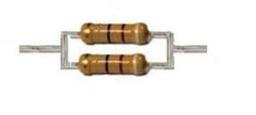Did you find an error or inaccuracy? Feel free to write us. Thank you!Tips to related online calculators
Looking for calculator of harmonic mean?
Looking for a statistical calculator?

## Related math problems and questions:

• ResistanceA resistor having an electrical resistance of 1.5 k ohms passes an electrical current of 0.1 A. Calculate what voltage is between the terminals of the resistor.
• Resistors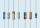Two resistors connected in series give the resulting resistance 245Ω and 60Ω in parallel. Determine the resistance of these resistors.
• R1+R2 in parallelThe resistance ratio of the first and second resistors is 3: 1 and the current flowing through the first resistor is 300 mA. What current flows through the second resistor (resistors connected in parallel)?
• Resistance - ohmsIf the resistance of the first resistor is 300 ohms and the resistance of the second resistor is 100 ohms. What is the resulting resistance?
• ResistanceDetermine the resistance of the bulb with the current 200 mA and is in a regular lamp (230V).
• Closed circuitIn a closed circuit, there is a voltage source with U1 = 12 V and with an internal resistance R1 = 0.2 Ω. The external resistance is R2 = 19.8 Ω. Determine the electric current and terminal voltage.
• Serial resistors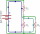In the circuit shown ( two resistiors R1 and R2 serial connected), the total resistance from A to B is 16 ohms. Find the resistance R2 and the current through the R1 = 12-Ohm resistor, if voltage between A and B is 10 V.
• Three resistors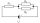You have to connect three identical resistors with 12 Ohm resistances so that you gradually get the resulting resistance a) 36 Ohm, b) 4 Ohm, 18 Ohm. Draw a diagram of each circuit.
• The resistanceWhat is the resistance of an aluminum wire 0.2 km long and 10 mm in diameter?
• Two resistors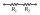Two resistors when they give 25 ohms in series and 4 ohms in parallel what the values of
• Two resistorsTwo resistors 20 Ω and 60 Ω are connected in series and an external voltage of 400 V is connected to them. What are the electrical voltages on the respective resistors? Please comment!
• Current in the conductorCalculate the current in the conductor (in mA) if it is connected to a 4.5 V voltage source and its resistance is 20 (ohm).
• Circuit voltageCalculate the closed-circuit voltage. Calculate the power on the resistors. R1 = 30 ohms, R2 = 10 ohms. I = 0.1A. U =?. The resistors are connected in series, one after the other.
• Resistor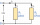Resistor 1 with a resistance of 100 Ohms and resistor 2 with a resistance of 400 Ohms are connected side by side in the circuit. There is a voltage of 80V between the resistor terminals. a) Draw a circuit diagram and write the entered quantities in it b)
• Serial connectionIf the voltage on the first resistor is 6 V and the resistance ratio of the first and second resistors is 1: 3, what will be the voltage across the second resistor if they are connected in series?
• Mains voltageHow much electric current flows through the appliance with a resistance of 40kΩ, which is connected to the mains voltage (230 V)?
• Reciprocal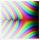What is the reciprocal of the sum of the reciprocals of 7 and 6?